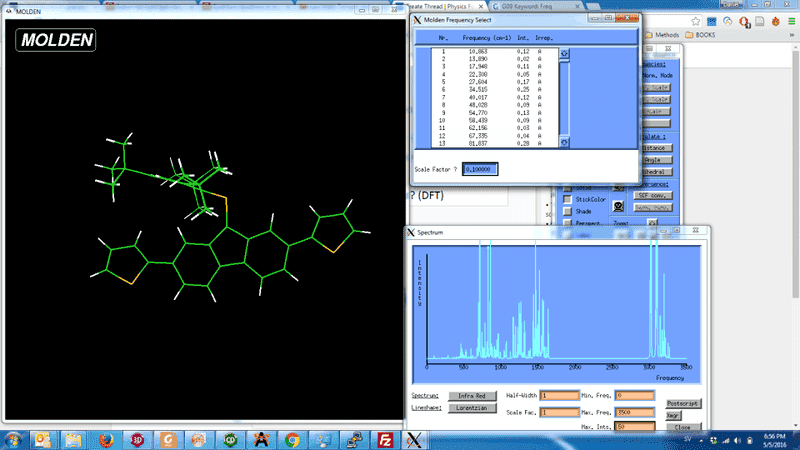# How to find vibrational frequency for specific bond? (DFT)

• A
• Dan Zar

#### Dan Zar

Hello,
I performed an optimization and frequency calculation at the ub3lyp 6-311g level of theory. The compound has the formula C39H41PS2. Using Molden, I am having a hard time identifying the frequency corresponding to the stretching of a phosphorus carbon double bond present in the molecule. I expect the frequency to be very low in intensity which makes my task harder. I am wondering if there is any way to obtain this information directly from the output file without having to click every frequency on Molden. Thank you very much.The calculation does not give you a value for the stretching of only this bond. Rather this stretching will contribute to several normal modes. Looking at the output of your calculation, you should see a list of the normal vibrations and the contribution of the individual atoms. Identify those normal modes where the C and P atom in question make a large contribution.

## 1. How does DFT calculate the vibrational frequency for a specific bond?

DFT (Density Functional Theory) calculates the vibrational frequency for a specific bond by solving the Schrödinger equation and using the Hessian matrix, which contains information about the potential energy surface of the molecule.

## 2. What is the role of the Hessian matrix in calculating vibrational frequency using DFT?

The Hessian matrix, also known as the force constant matrix, contains information about the second derivatives of the molecule's potential energy with respect to the atomic coordinates. This information is used to calculate the vibrational frequencies of the molecule.

## 3. How accurate is DFT in predicting vibrational frequencies for specific bonds?

DFT has been shown to be accurate in predicting vibrational frequencies for specific bonds, with an average error of less than 5%. However, the accuracy can vary depending on the specific functional and basis set used.

## 4. Can DFT be used for all types of bonds?

Yes, DFT can be used for all types of bonds, including single, double, and triple bonds. It can also be used for non-bonded interactions such as hydrogen bonding.

## 5. Are there any limitations to using DFT for calculating vibrational frequencies?

While DFT is a powerful tool for predicting vibrational frequencies, it does have some limitations. One limitation is that it assumes the molecules are in their ground state, so it may not accurately predict frequencies for excited states. Additionally, the accuracy of DFT can be affected by the choice of functional and basis set, as well as the size of the molecule being studied.